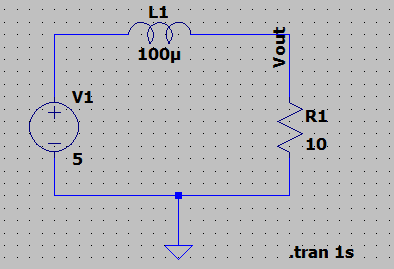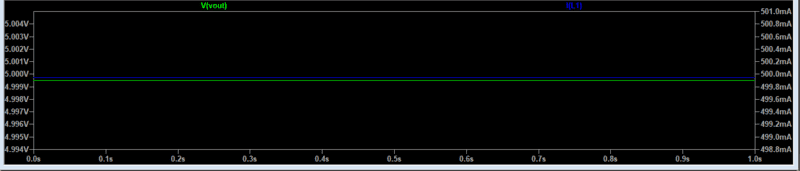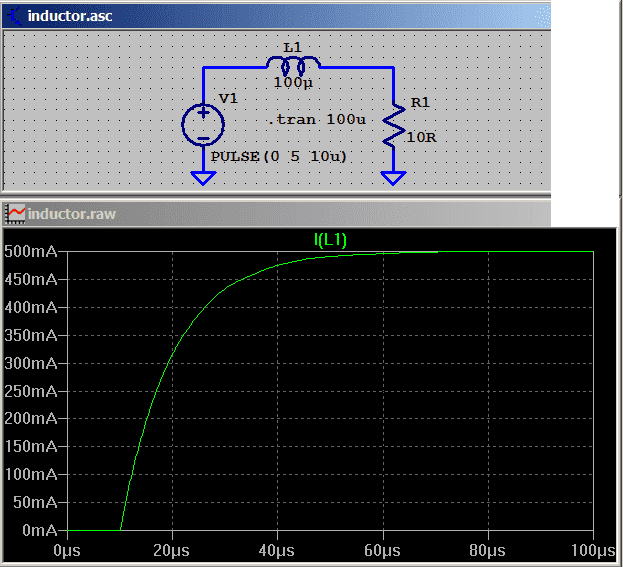# Analysis of Inductor Circuit

• PhysicsTest

#### PhysicsTest

TL;DR Summary
To understand the inductor circuit.
I am trying to understanding the inductor circuit. I have drawn the below circuit and try to simulateThe Inductor current comes to 500mA and Vout is 5V as per simulation. The current in inductor should slowly increase from 0A to 500mA but i cannot see that in the waveform. Is my understanding correct?

but i cannot see that in the waveform
Neither can we. What waveform ?
Do you have some equations to describe the behaviour of your circuit ?

##\ ##

•PhysicsTest
The time constant for this circuit is L1/R1 = 10uSec.

•berkemanThis is the output waveform i can get. Ok now i started writing the equation for the circuit.
##i(t) = \frac{V} {R} (1 - e^{\frac{-Rt} L})##
Substituting the values
##i(1) = 5/10*(1 - e^{-10^5}) ##
##i = 0.5A##. The answer is matching.

•BvU
So zoom into the first few us of the transient waveform to see the LR time constant...

The DC analysis sets up 500 mA before the run.
You must use; V1 pulse; initial=0V; Von=5V; Tdelay=5usec;
and .TRAN stop time = 100 usec.
Then see the current rise over about 50 usec.Last edited:
••BvU, PhysicsTest and berkeman